# AP Board 5th Class Maths Solutions 8th Lesson Fractions

Andhra Pradesh AP Board 5th Class Maths Solutions 8th Lesson Fractions Textbook Exercise Questions and Answers.

## AP State Syllabus 5th Class Maths Solutions Chapter 8 Fractions

I. Observe the following table.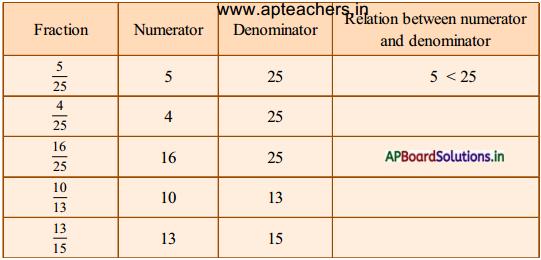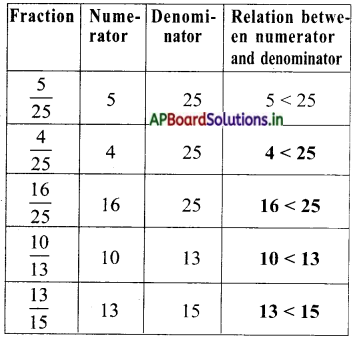What do you observe from the above table?
Here, in all fractions the numerator is less than the denominator.II. If Hema and Gopi got 7 and 9 biscuits then complete this table.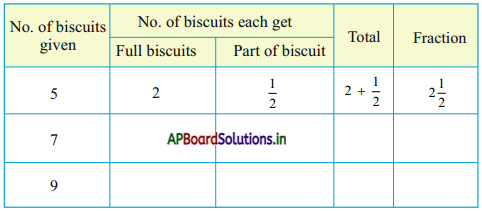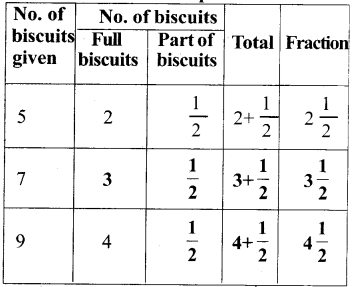III. Observe the fractions : $$\frac{3}{4}, \frac{4}{5}, \frac{6}{7}, \frac{12}{13}, \frac{25}{28}$$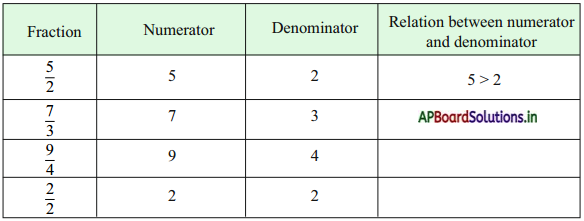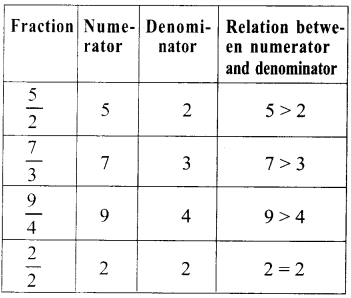What do you say?
Here is all fractions have numerator is greater than or equal to denominator. These types of fractions are called improper fractions.Do this: (TextBook Page No.129)

Question 1.
Write any 5 proper fractions.
Proper fractions: $$\frac{3}{4}, \frac{4}{5}, \frac{6}{7}, \frac{12}{13}, \frac{25}{28}$$

Question 2.
Write any 5 1m proper fractions.
Improper fractions: $$\frac{7}{6}, \frac{26}{22}, \frac{21}{20}, \frac{28}{25}, \frac{13}{12}$$

Question 3.
Write any 5 mixed fractions.
Mixed fractions: $$3 \frac{2}{3}, 7 \frac{1}{2}, 9 \frac{3}{5}, 8 \frac{2}{3}, 6 \frac{5}{7}$$

Question 4.
Convert these fraction into mixed fraction $$\frac{5}{2}, \frac{7}{3}, \frac{9}{4}, \frac{11}{2}$$
Conversion of fraction into mixed fraction.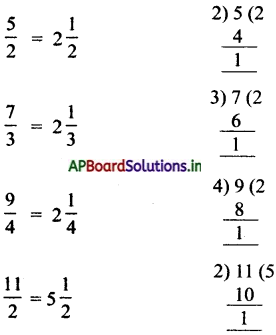Question 5.
Convert these fractions into improper fraction $$4 \frac{2}{3}, 5 \frac{3}{4}, 6 \frac{2}{5}, 3 \frac{1}{2}$$.
Conversion of Mixed fractions into improper fractions

$$4 \frac{2}{3}=\frac{3 \times 4+2}{3}=\frac{14}{3}$$ $$5 \frac{3}{4}=\frac{4 \times 5+3}{4}=\frac{23}{4}$$ $$6 \frac{2}{5}=\frac{5 \times 6+2}{5}=\frac{32}{5}$$

$$3 \frac{1}{2}=\frac{2 \times 3+1}{2}=\frac{7}{2}$$.Do this: (TextBook Page No. 132)

Question 1.
Write any three equivalent fractions to the given fractions.
a) $$\frac{4}{8}$$
b) $$\frac{1}{3}$$
c) $$\frac{3}{7}$$
d) $$\frac{20}{24}$$
a) Equivalent fractions to $$\frac{4}{8}=\frac{8}{16}=\frac{12}{24}=\frac{16}{32}$$
b) Equivalent fractions to $$\frac{1}{3}=\frac{3}{9}=\frac{2}{6}=\frac{4}{12}$$
c) Equivalent fractions to $$\frac{3}{7}=\frac{9}{21}=\frac{6}{14}=\frac{12}{28}$$
d) Equivalent fractions to $$\frac{20}{24}=\frac{40}{48}=\frac{60}{72}=\frac{80}{96}$$

Exercise 1:

Question 1.
Simplify the following fractions. (by cancellation method).
(i) $$\frac{105}{15}$$
(ii) $$\frac{200}{20}$$
(iii) $$\frac{7}{10}$$
(iv) $$\frac{666}{66}$$
(v) $$\frac{125}{1000}$$
(vi) $$\frac{120}{200}$$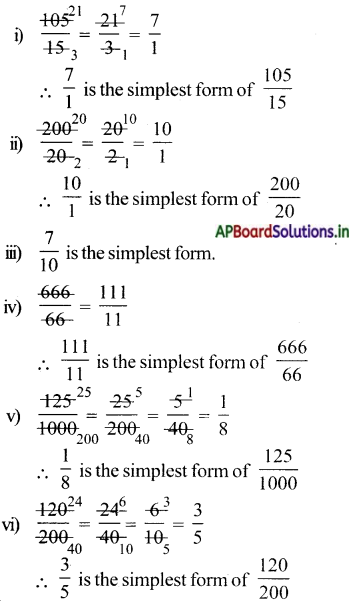Question 2.
Simplify the following fractions. (by H.C.F. method)
(i) $$\frac{12}{18}$$
(ii) $$\frac{14}{35}$$
(iii) $$\frac{22}{55}$$
(iv) $$\frac{27}{36}$$
(v) $$\frac{128}{164}$$
(vi) $$\frac{210}{427}$$
(i) HCF of 12 and 18 is 6.
$$\frac{12 \div 6}{18 \div 6}=\frac{2}{3}$$
So, $$\frac{2}{3}$$ is the simplest form of $$\frac{12}{18}$$.

(ii) HCF of 14 and 35 is 7.
$$\frac{14 \div 7}{35 \div 7}=\frac{2}{5}$$
So, $$\frac{2}{5}$$ is the simplest form of $$\frac{14}{35}$$.

(iii) HCF of 22 and 55 is 11.
$$\frac{22 \div 11}{55 \div 11}=\frac{2}{5}$$
So, $$\frac{2}{5}$$ is the simplest form of $$\frac{22}{55}$$.

(iv) HCF of 27 and 36 is 9.
$$\frac{27 \div 9}{36 \div 9}=\frac{3}{4}$$
So, $$\frac{3}{4}$$ is the simplest form of $$\frac{27}{36}$$.

(v) HCF of 128 and 164 is 4.
$$\frac{128 \div 4}{164 \div 4}=\frac{32}{41}$$
So, $$\frac{32}{41}$$ is the simplest form of $$\frac{128}{164}$$.

(vi) HCF of 210 and 427 is 7.
$$\frac{210 \div 7}{427 \div 7}=\frac{30}{61}$$
So, $$\frac{30}{61}$$ is the simplest form of $$\frac{210}{427}$$.

Question 3.
Convert the following fractions into the simplest form by both the methods.
(i) $$\frac{16}{64}$$
(ii) $$\frac{12}{18}$$
(iii) $$\frac{30}{50}$$
(iv) $$\frac{40}{25}$$
(v) $$\frac{16}{32}$$
(vi) $$\frac{8}{40}$$
(i) 1s tMethod: HCF of 16 and 64 is 4.
$$\frac{16 \div 4}{64 \div 4}=\frac{4}{16}=\frac{1}{4}$$
So, $$\frac{1}{4}$$ is the simplest form of $$\frac{16}{64}$$.

2nd Method =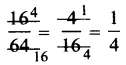(ii) 1st Method HCF of 12 and 28 is 4.
$$\frac{12 \div 4}{28 \div 4}=\frac{3}{7}$$,
So $$\frac{3}{5}$$ is simplest form of $$\frac{12}{28}$$ .
2nd Method: =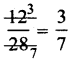iii) 1st Method : HCF of 30 and 50 is 10.
$$\frac{30 \div 10}{50 \div 10}=\frac{3}{5}$$
So, $$\frac{3}{5}$$ is simplest form of $$\frac{30}{50}$$
2nd method $$\frac{30}{50}=\frac{3}{5}$$.

(iv) 1st method: HCF of 40 and 25 is 5
$$\frac{40 \div 5}{25 \div 5}=\frac{8}{5}$$
So $$\frac{8}{5}$$ is simplest form of $$\frac{40}{25}$$
2nd method: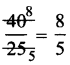(v) 1st method: HCF of 16 and 32 is 16.
$$\frac{16+16}{32+16}=\frac{1}{2}$$,
So $$\frac{1}{2}$$ is the simplest form of $$\frac{16}{32}$$.
2nd method: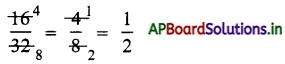vi) 1st method: HCF of 8 and 40 is 8.
$$\frac{8 \div 8}{40 \div 8}=\frac{1}{5}$$,
So $$\frac{1}{5}$$ is simplest form of $$\frac{8}{48}$$.
2nd method: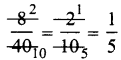Question 4.
To get equivalent fractions what should we do a given fraction ?
To get equivalent fractions, we multiply / divide both numerator and denominator by the same number.

Question 5.
Write any three equivalent fractions to the given fractions.
(i) $$\frac{5}{8}$$
(ii) $$\frac{32}{64}$$
(iii) $$\frac{3}{7}$$
(iv) $$\frac{125}{255}$$
(v) $$\frac{7}{10}$$
(i) Equivalent fractions to $$\frac{5}{8}$$ is $$\frac{10}{16}, \frac{15}{24} \text { and } \frac{20}{32}$$
(ii) Equivalent fractions to $$\frac{1}{2}, \frac{2}{4}, \frac{4}{8}, \frac{8}{16}$$
(iii) Equivalent fractions to $$\frac{3}{7}$$ is $$\frac{6}{14}, \frac{9}{21}, \frac{12}{28$$
(iv) Equivalent fractions to $$\frac{125}{255}$$ is $$\frac{5}{9}, \frac{25}{45} \text { and }$$
(v) Equivalent fractions to $$\frac{7}{10}$$ is $$\frac{14}{20}, \frac{21}{30}$$ and $$\frac{35}{50}$$

Question 6.
Govindamma distributed her 4 acrse of land to her 3 sons, then write the part of land each got in the form of a fraction.
Total land = 4 acres
No. of sons = 3
Part of land each got in the form of fraction = $$\frac{4}{3}$$ = 1$$\frac{1}{3}$$.Do these : (TextBook Page No.137)

Question 1.
Observe the example and write the correct fractions in the other circles.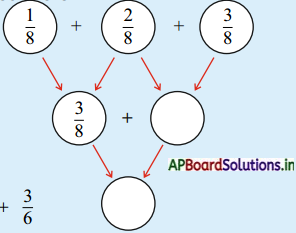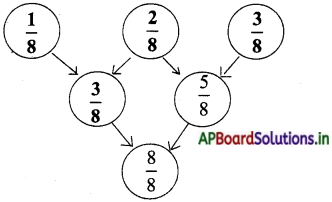Question 2.
Find the sum.
(i) $$\frac{2}{10}+\frac{4}{10}$$
(ii) $$\frac{2}{6}+\frac{3}{6}$$
(iii) $$1 \frac{1}{4}+3 \frac{1}{4}$$
(iv) $$2 \frac{1}{5}+3 \frac{1}{5}$$
(i) $$\frac{2}{10}+\frac{4}{10}=\frac{2+4}{10}=\frac{6}{10}$$
(ii) $$\frac{2}{6}+\frac{3}{6}=\frac{2+3}{6}=\frac{5}{6}$$
(iii) $$1 \frac{1}{4}+3 \frac{1}{4}=\frac{5}{4}+\frac{13}{4}=\frac{18}{4}$$
(iv) $$2 \frac{1}{5}+3 \frac{1}{5}=\frac{11}{5}+\frac{16}{5}+\frac{27}{5}$$

Question 3.
$$\frac{1}{2}$$ kg of a sugar packet, $$\frac{3}{6}$$ kg of jaggery are in a bag. Then what is the
total weight of two items in the bag?
Weight of sugar packet = $$\frac{1}{2}$$ kg
Weight ofjaggery packet = $$\frac{3}{6}$$ kg = $$\frac{1}{2}$$ kg
Total weight of two items = $$\frac{1}{2}$$ + $$\frac{3}{6}$$
$$\frac{3}{6}+\frac{3}{6}=\frac{3+3}{6}=\frac{6}{6}$$ = 1.Question 4.
Sakru paints $$\frac{1}{5}$$ th part of a wall on first day. $$\frac{2}{5}$$ th part of the wall on second day Then how much part lie painted in both the days?
Painting part of wall on first day = $$\frac{1}{5}$$
Painting part of wall on second day = $$\frac{2}{5}$$
Painting part of wall on both the days = $$\frac{1}{5}+\frac{2}{5}=\frac{1+2}{5}=\frac{3}{5}$$

Question 5.
Polamma had some money. She spent $$\frac{3}{6}$$th part of money on books. $$\frac{1}{6}$$ th part of money on pens, pencils and erasers. Then how much part money did she spend ¡n total?
Money spent on books = $$\frac{3}{6}$$th part
Money spent on pens = $$\frac{1}{6}$$th part
pencils and erasers.
Money spent in total = $$\frac{3}{6}+\frac{1}{6}=\frac{3+1}{6}$$ = $$\frac{4}{6}$$th part

Do these: (TextBook Part No. 139)

Question 1.
Complete this.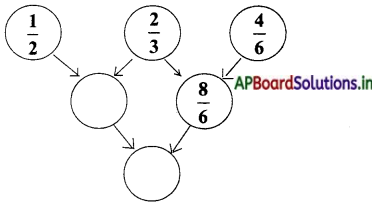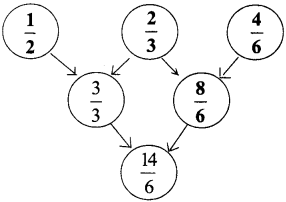Question 2.
Find the sum.
(i) $$\frac{1}{5}+\frac{3}{4}$$
(ii) $$\frac{3}{4}+\frac{5}{6}$$
(iii) $$1 \frac{2}{3}+2 \frac{5}{6}$$
(iv) $$3 \frac{1}{8}+2 \frac{5}{6}$$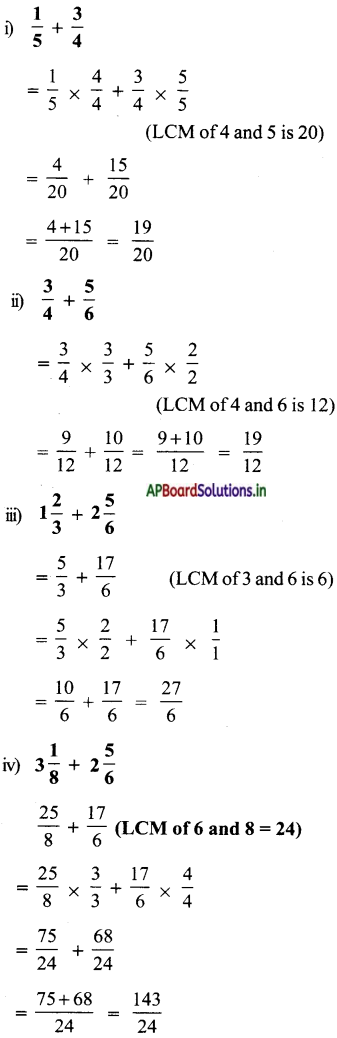Question 3.
Seetamma read $$\frac{1}{5}$$ th part of a book on Monday, $$\frac{4}{10}$$ part of the book on Tuesday. Then how much part did she complete on two days ?
Book read on Monday = $$\frac{1}{5}$$ th part
Book read on Tuesday = $$\frac{4}{10}$$ th part
Book read on two days = $$\frac{1}{5}$$ + $$\frac{4}{10}$$
LCM of 5 and 10 is 10.
= $$\frac{1}{5} \times \frac{2}{2}+\frac{4}{10} \times \frac{1}{1}$$
= $$\frac{2}{10}+\frac{4}{10}=\frac{2+4}{10}=\frac{6}{10}$$

Question 4.
Polayya painted a wall of $$\frac{3}{4}$$ th part on 1st day and $$\frac{3}{6}$$ th part of the wall on 2nd day. Then how much part he painted the wall in two days ?
Painted part of wal on 1 st day = $$\frac{1}{5}$$
Painted part of wall on 2nd day = $$\frac{3}{6}$$
Painted part in two days = $$\frac{1}{5}$$ + $$\frac{3}{6}$$
LCM of 5 and 6 is = 30
= $$\frac{1}{5} \times \frac{6}{6}+\frac{3}{6} \times \frac{5}{5}=\frac{21}{30}=\frac{7^{t h}}{10} \text { Part }$$

Try this: (TextBook Part No.139)

Question 1.
Add $$5 \frac{6}{8}+4 \frac{1}{7}$$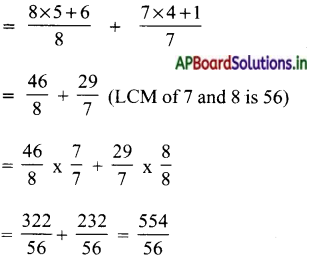Do this: (TextBook Page No.141)

Question 1.
Complete this: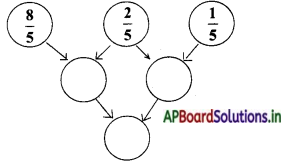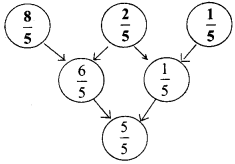Question 2.
Do the following.
(i) $$\frac{6}{10}-\frac{1}{10}$$
(ii) $$\frac{3}{15}-\frac{1}{15}$$
(iii) $$1 \frac{3}{15}-1 \frac{1}{15}$$
(iv) $$2 \frac{4}{7}-1 \frac{2}{7}$$
(i) $$\frac{6}{10}-\frac{1}{10}$$
= $$\frac{6-1}{10}=\frac{5}{10}$$

(ii) $$\frac{3}{15}-\frac{1}{15}$$
= $$\frac{3-1}{15}-\frac{2}{15}$$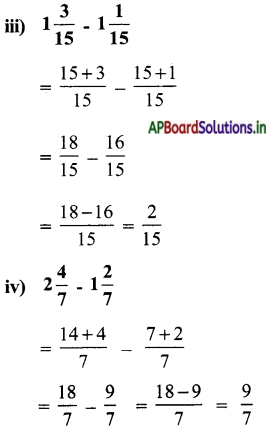Question 3.
Eswar painted $$\frac{1}{6}$$ th part of a wall on first day. Then how much part will remain to complete?
Painted part ofwal on Sunday = $$\frac{1}{6}$$th part
Total part = 1
Remaining part to complete = $$\frac{1}{1}-\frac{1}{6}$$
LCM of 1 and 6 is 6.
= $$\frac{1}{1} \times \frac{6}{6}-\frac{1}{6} \times \frac{1}{1}=\frac{6}{6}-\frac{1}{6}$$
= $$\frac{6-1}{6}-\frac{5}{6}$$.

Question 4.
Gown completed $$\frac{1}{4}$$ part of her homework on Sunday. $$\frac{5}{12}$$ part on Sunday morning. How much part did she complete? How much part of home work is left?
Work complete on Saturday = $$\frac{1}{4}$$
Work completed on Sunday = $$\frac{5}{12}$$
Work completed both days = $$\frac{1}{4}$$ + $$\frac{5}{12}$$
LCM of 4 and 12 is 12.
= $$\frac{1}{4} \times \frac{3}{3}+\frac{5}{12} \times \frac{1}{1}$$
= $$\frac{3}{12}+\frac{5}{12}=\frac{3+5}{12}=\frac{8}{12}$$
Remainig part left = $$\frac{1}{1}-\frac{8}{12}$$
LCM of 1 and 12 is 12
= $$\frac{1}{1} \times \frac{12}{12}-\frac{8}{12} \times \frac{1}{1}$$
= $$\frac{12}{12}-\frac{8}{12}=\frac{12-8}{12}=\frac{4}{12}=\frac{1}{3} \text { th part }$$

Try these: (TextBook Part No.142)

Question 1.
Complete this: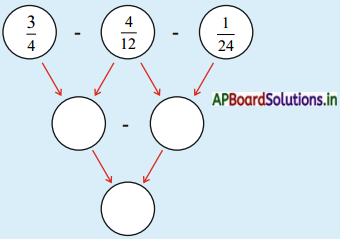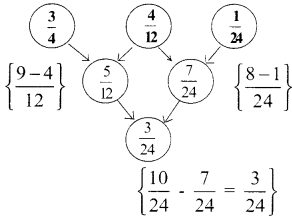Exercise 2:

Question 1.
Do the following:
a) $$\frac{3}{4}+\frac{7}{4}$$
b) $$1 \frac{1}{2}$$
c) $$\frac{8}{3}+\frac{2}{5}$$
d) $$\frac{6}{3}+\frac{7}{4}$$
e) $$\frac{3}{5}+\frac{9}{11}$$
f) $$\frac{10}{10}+\frac{5}{20}$$
g) $$\frac{9}{10}+\frac{4}{15}$$
h) $$\frac{5}{20}+\frac{13}{30}$$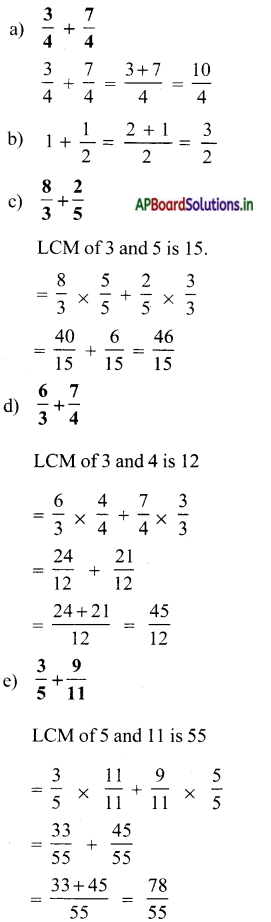Question 2.
Do the following.
a) $$\frac{3}{7}-\frac{1}{7}$$
b) 6 – $$\frac{1}{3}$$
c) $$\frac{3}{8}-\frac{3}{16}$$
d) $$\frac{8}{7}-\frac{5}{8}$$
e) $$\frac{8}{7}-\frac{5}{8}$$
f) $$\frac{13}{15}-\frac{7}{20}$$
g) $$\frac{63}{40}-\frac{9}{10}$$
h) $$\frac{7}{15}-\frac{3}{10}$$
a) $$\frac{3}{7}-\frac{1}{7}$$
= $$\frac{3-1}{7}-\frac{2}{7}$$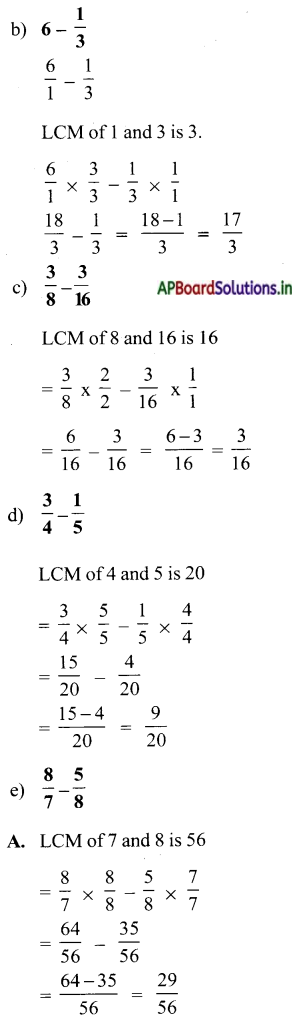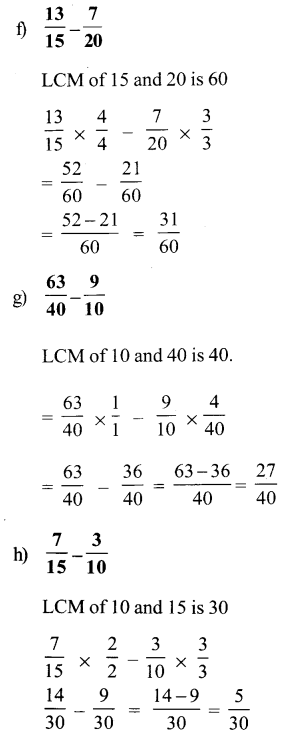Question 3.
Find the difference between 5$$\frac{1}{3}$$ and 2$$\frac{4}{7}$$
$$\frac{15+1}{3}-\frac{14+4}{7}=\frac{16}{3}-\frac{18}{7}$$
LCM of 3 and 7 = 21
$$\frac{16}{3} \times \frac{7}{7}-\frac{18}{7} \times \frac{3}{3}$$
= $$\frac{112}{21}-\frac{54}{21}=\frac{58}{21}$$

Question 4.
Seetha purchased 1$$\frac{1}{2}$$ litre of sunflower oil, $$\frac{3}{4}$$ litre of groundnut oil. How much of oil she purchased in total?
Purchased quantity of sunflower oil = 1$$\frac{1}{2}$$ litr.
Purchased quantity of ground nut oil = $$\frac{3}{4}$$ litr.
Purchased quantity of total oil = 1 $$\frac{1}{2}$$ + $$\frac{3}{4}$$
= $$\frac{3}{2}$$ + $$\frac{3}{4}$$
LCM of 2, 4 is 4
= $$\frac{3}{2} \times \frac{2}{2}+\frac{3}{4} \times \frac{1}{1}$$
= $$\frac{6}{4}+\frac{3}{4}=\frac{6+3}{4}=\frac{9}{4}$$

Question 5.
Vimala purchased 1 $$\frac{3}{4}$$ m of cotton cloth for skirt, $$\frac{3}{4}$$ m of cloth for blouse. How much cloth is purchased by her?
Purchased cloth for skirt = 1 $$\frac{3}{4}$$ m
Purchased cloth for blouse = $$\frac{3}{4}$$ m
Purchased cloth Total = 1$$\frac{3}{4}$$ + $$\frac{3}{4}$$
= $$\frac{7}{4}$$ + $$\frac{3}{4}$$ = $$\frac{10}{4}$$ m.Question 6.
A water tank is filled with $$\frac{9}{10}$$th part of water, but $$\frac{3}{5}$$ th part of water is consumed in a day. Then find the remaining part of water in the tank?
Total quantity of water infilled in tank = $$\frac{9}{10}$$ th part
Consumed quantity of water is = $$\frac{3}{5}$$ th part
Remaining part of water in the tank = $$\frac{9}{10}-\frac{3}{5}=\frac{9-6}{10}=\frac{3}{10} \text { th part }$$

Do these: (TextBook Page No.145)

Question 1.
Four hundred and eighty five (point) two six seven.

Question 2.
Write the place value of all digits in 293.819
Given numbers = 293.819
place valu e of 2 = 200
place value of 9 = 90
place value of 5 = 5
place value of 8 = $$\frac{1}{80}$$
place value of 1 = $$\frac{1}{900}$$
place value of 9 = $$\frac{9}{1000}$$

Question 3.
Write any 5 examples for decimal fractions.
(i) $$\frac{4756}{100}$$ = 47.56
(ii) $$\frac{87685}{1000}$$ = 87.685
(iii) $$\frac{763407}{1000}$$ = 763.407
(iv) $$\frac{86734}{10000}$$ = 8.6734
(v) $$\frac{96302}{10}$$= 9630.2Exercise 3:

Question 1.
Fill in the blanks:

a) In improper fraction, numerator is ………………… than the denominator.
greater

b) $$\frac{6}{6}$$ is ………………… fraction (which type?)
improper

c) 3$$\frac{1}{2}$$ is ………………… fraction (which type?)
mixed

d) $$\frac{9}{6}$$ ………………… is fraction (which type?)
improper

e) $$\frac{2}{5}$$ is ………………… fraction (which type?)
proper

f) A function having whole number and proper fraction is called ………………… fraction.
(mixed)

Question 2.
Convert $$\frac{9}{6}$$ into mixed fraction.
Mixed fraction of $$\frac{9}{6}=1 \frac{3}{6}$$.

Question 3.
Convert 2$$\frac{1}{5}$$ into an improper fraction.
Improper fraction of $$2 \frac{1}{5}=\frac{2 \times 5+1}{5}=\frac{11}{5}$$.Question 4.
Write any 5 equivalent fractions to $$\frac{2}{3}$$.
Equivalent fractions of $$\frac{2}{3}$$ is $$\frac{4}{6}, \frac{6}{9}, \frac{8}{12}, \frac{10}{15}$$ and $$\frac{16}{18}$$.

Question 5.
Write simplest form of fraction for $$\frac{25}{75}$$.
Cancilation method: =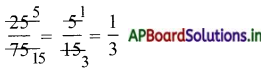∴ Simplest form of $$\frac{25}{75}$$ is $$\frac{1}{3}$$.

Question 6.
Write two equivalent fractions to $$\frac{64}{36}$$.
Equivalent fractions to $$\frac{64}{36}$$
= $$\frac{64 \div 2}{36 \div 2}=\frac{32}{18}$$
= $$\frac{64 \div 4}{36 \div 4}=\frac{16}{9}$$.

Question 7.
Classify the following as like and unlike frations.
$$\frac{3}{5}, \frac{2}{7}, \frac{8}{5}, \frac{9}{5}, \frac{8}{4}, \frac{1}{5}$$
Like fractions: $$\frac{1}{5}, \frac{3}{5}, \frac{8}{5}, \frac{9}{5}$$
Unlike fractions = $$\frac{2}{7}, \frac{8}{4}$$.Question 8.
Fill in the blanks.
a) $$\frac{15}{20}=\frac{3}{\square}$$
b) $$\frac{2}{5}=\frac{\square}{50}$$
c) $$\frac{3}{5}=\frac{\square}{30}$$
a) $$\frac{15}{20}=\frac{3}{4}$$
b) $$\frac{2}{5}=\frac{20}{50}$$
c) $$\frac{3}{5}=\frac{18}{30}$$

Question 9.
Fill in the blanks with = or ≠ (≠ denotes not equal to)
a) $$\frac{1}{2}$$ ____ $$\frac{8}{16}$$
b) $$\frac{9}{15}$$ ____ $$\frac{27}{30}$$
c) $$\frac{6}{13}$$ ____ $$\frac{12}{39}$$
a) $$\frac{1}{2}$$ = $$\frac{8}{16}$$
b) $$\frac{9}{15}$$ ≠ $$\frac{27}{30}$$
c) $$\frac{6}{13}$$ ≠ $$\frac{12}{39}$$

Question 10.
Fill in the blanks with equivalent fractions.
a) $$\frac{1}{2}$$ —- $$\frac{8}{16}$$ ____, ____, ____
a) $$\frac{1}{2}$$ —- $$\frac{8}{16} \frac{2}{4}, \frac{3}{6}, \frac{5}{10}$$.

Question 11.
a) $$\frac{6}{5}+\frac{1}{5}$$ = ———-
b) $$\frac{5}{7}+\frac{2}{14}$$ = ———-
c) $$\frac{15}{32}+\frac{3}{8}$$ = ———-
d) $$\frac{11}{16}+1 \frac{1}{8}$$ = ———-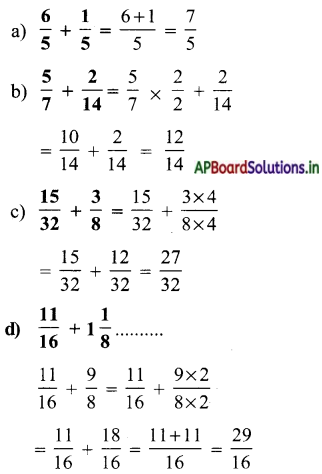Question 12.
Kavitha studied $$\frac{1}{2}$$ part of a book on 1st day, $$\frac{1}{3}$$ part on 2nd day. then how much part she studied in both the days ?
On I st day completed book part = $$\frac{1}{2}$$
On 2nd day completed book part = $$\frac{1}{3}$$
On both days completed book part = $$\frac{1}{2}$$ + $$\frac{1}{3}$$
LCM of 2 and 3 is 6.
= $$\frac{1}{2} \times \frac{3}{3}+\frac{1}{3} \times \frac{2}{2}$$
= $$\frac{3}{6}+\frac{2}{6}$$
= $$\frac{3+2}{6}=\frac{5}{6}$$.

Question 13.
Koushik went to school km by walk. He went on bicycle with his friend for the remaining distance $$\frac{3}{4}$$ km. Then find the distance to school from his house.
Distance covered by walk = $$\frac{1}{4}$$ km
Distance covered by bicycle = $$\frac{3}{4}$$ km
Total distance to school from his house = $$\frac{1}{4}$$ + $$\frac{3}{4}$$
= $$\frac{1+3}{4}=\frac{4}{4}$$ km

Question 14.
a) $$\frac{8}{10}-\frac{2}{10}$$ = ………………
b) $$\frac{1}{3}-\frac{1}{9}$$ = ………………
c) $$\frac{15}{32}-\frac{3}{8}$$ = ………………
d) $$6 \frac{1}{16}-1 \frac{1}{8}$$ = ………………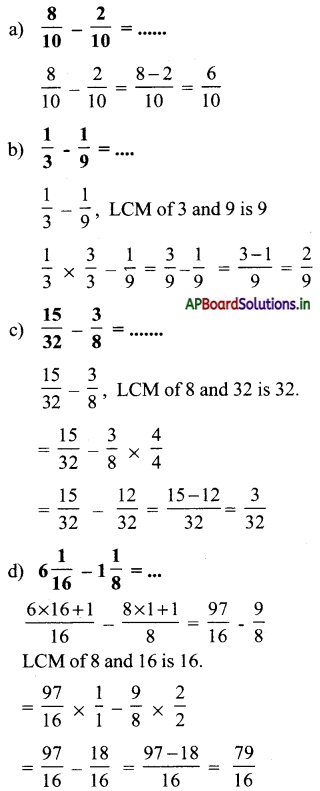Question 15.
$$\frac{2}{3}$$rd nd part of students in a school is boys. Find the part of girls.
Part of boys in a school = $$\frac{2}{3}$$rd
Part of girls inaschool= 1 – $$\frac{2}{3}$$.
= $$\frac{3-2}{3}=\frac{1}{3}$$rd.

Question 16.
Subtract $$\frac{21}{4}$$ from the total of $$\frac{7}{2}$$ and $$\frac{8}{3}$$.
Total of $$\frac{7}{2}$$ and $$\frac{8}{3}$$ = $$\frac{7}{2} \times \frac{3}{3}+\frac{8}{3} \times \frac{2}{2}$$
= $$\frac{21}{6}+\frac{16}{6}=\frac{21+16}{6}=\frac{37}{6}$$
Subtract of $$\frac{37}{6}-\frac{21}{4}$$ =
LCM of 6 and 4 is 12 = $$\frac{37}{6} \times \frac{2}{2}-\frac{21}{4} \times \frac{3}{3}=\frac{74-63}{12}=\frac{11}{12}$$

Question 17.
Govind studied $$\frac{2}{5}$$ th part of a book on 1st day, $$\frac{1}{7}$$ th part on 2nd day. Then how much part is yet to be completed?
Completed part of book on 1st day = $$\frac{2}{5}$$th
Completed part of book on 2nd day = $$\frac{1}{7}$$th
Completed part of book on two days = $$\frac{2}{5}$$ + $$\frac{1}{7}$$
LCM of 5 and 7 is 35.
= $$\frac{2}{5} \times \frac{7}{7}+\frac{1}{7} \times \frac{5}{5}$$
= $$\frac{14}{35}+\frac{5}{35}=\frac{14+5}{35}=\frac{19}{35}$$
remaining part of book yet to be completed = 1 – $$\frac{19}{35}$$
= $$\frac{1 \times 35}{35}-\frac{19}{35} \times \frac{1}{1}$$
= $$\frac{35}{35}-\frac{19}{35}=\frac{35-19}{35}=\frac{14}{35}$$Question 18.
Write in words 189.257
6 is in $$\frac{1}{100}$$th place.